Ex 5.7

Chapter 5 Class 12 Continuity and Differentiability
Serial order wise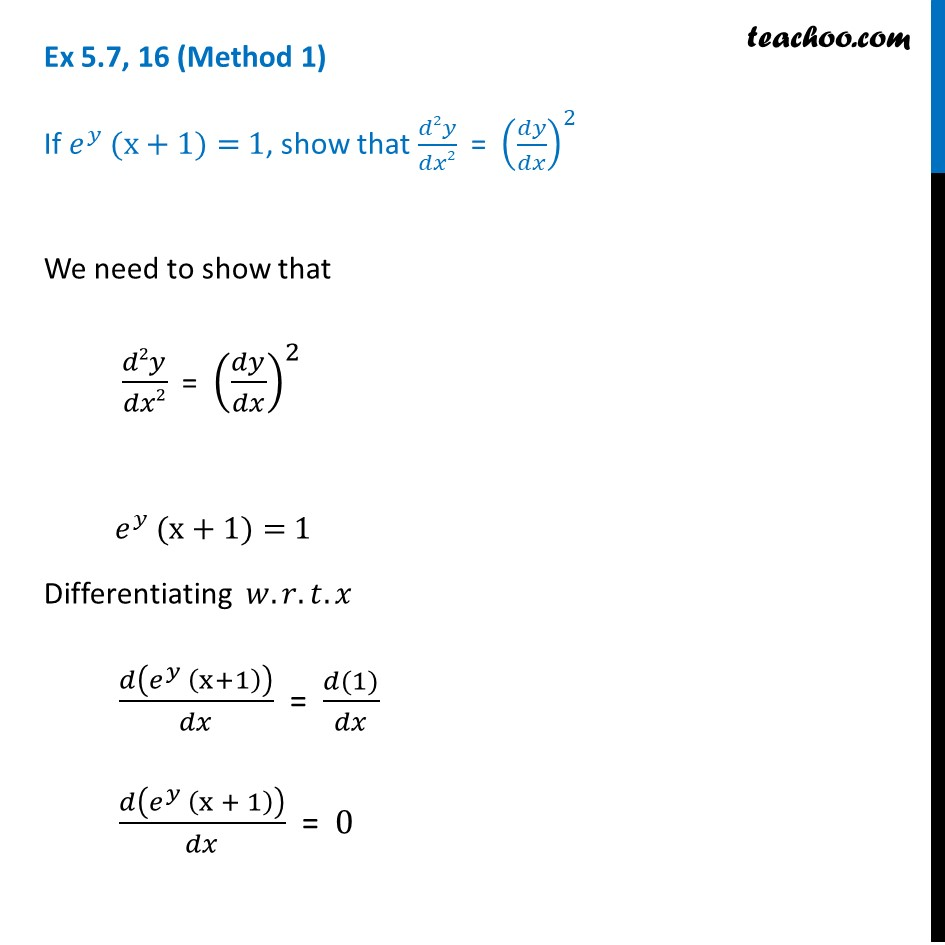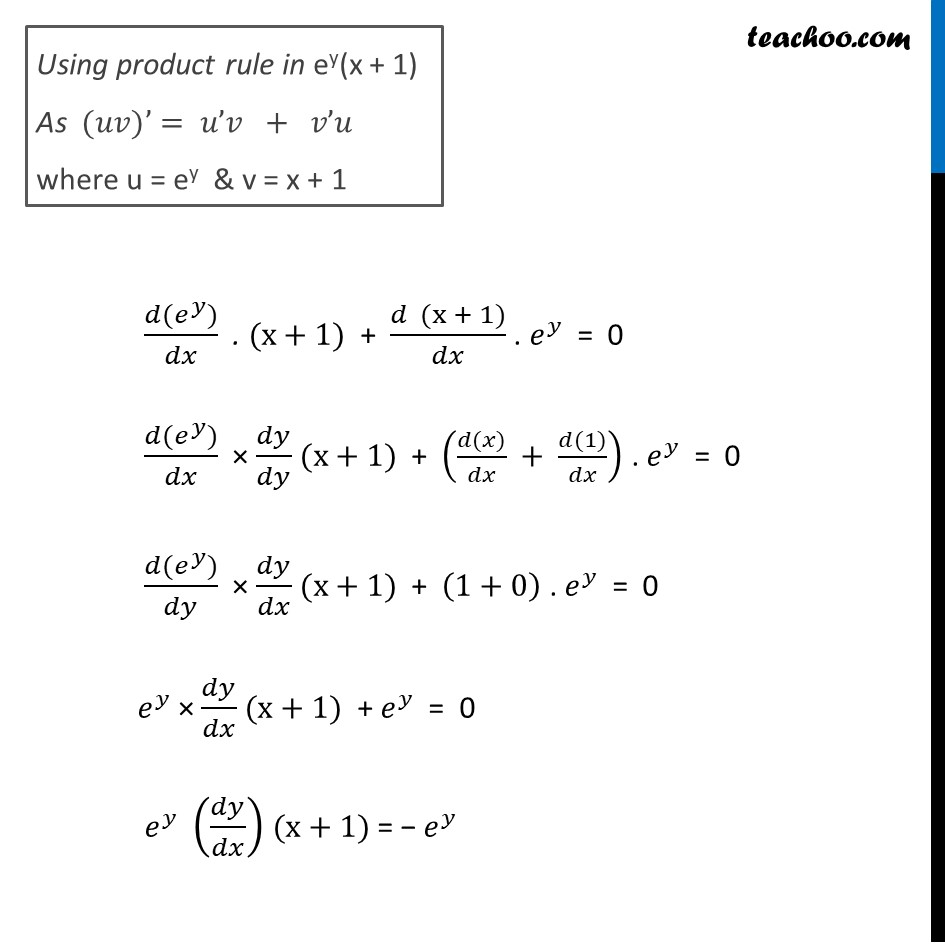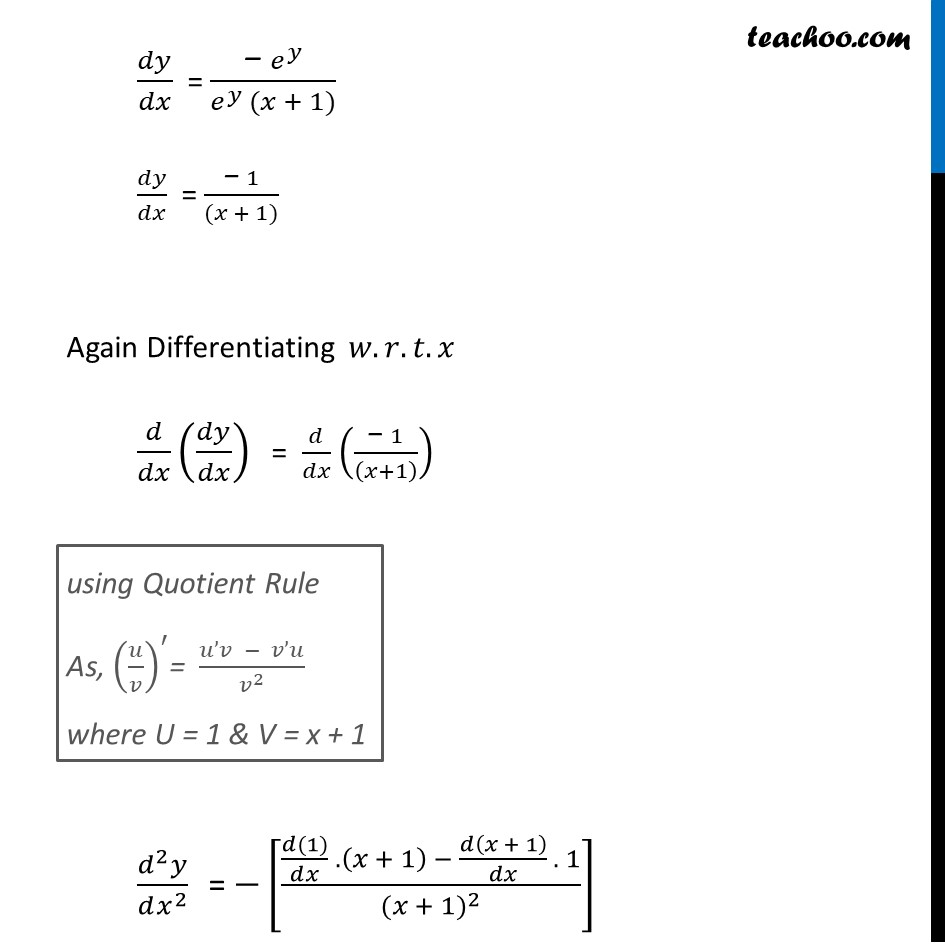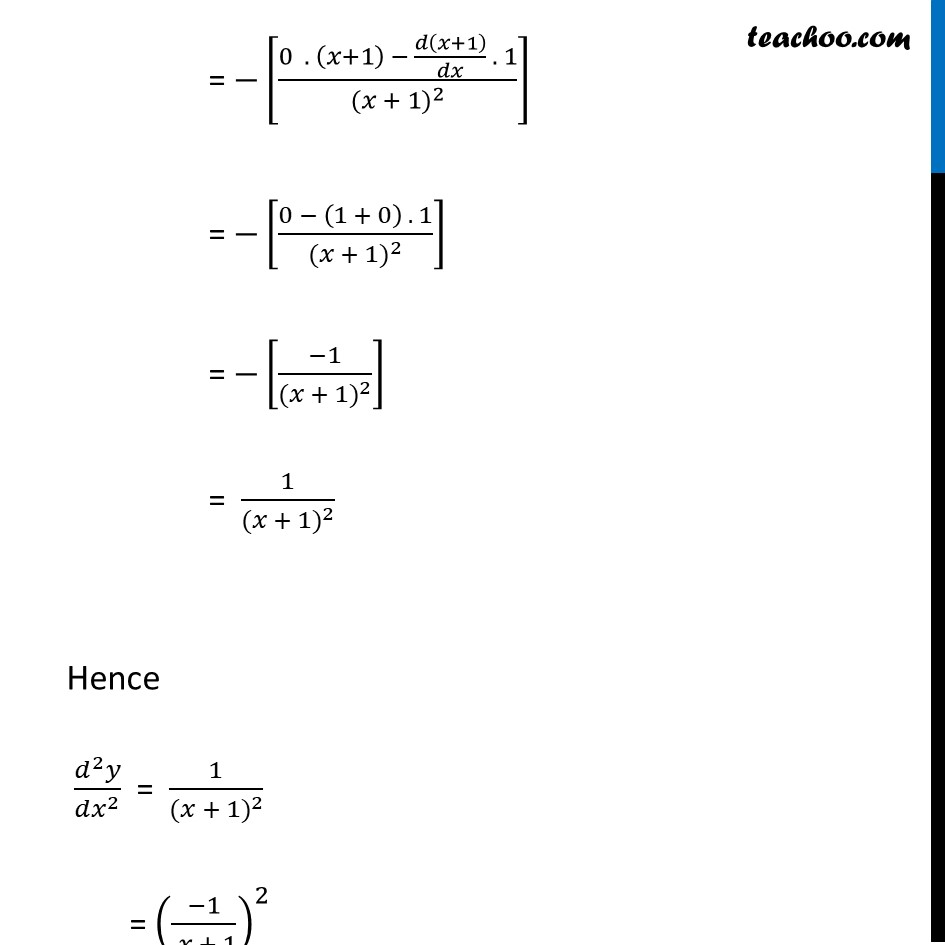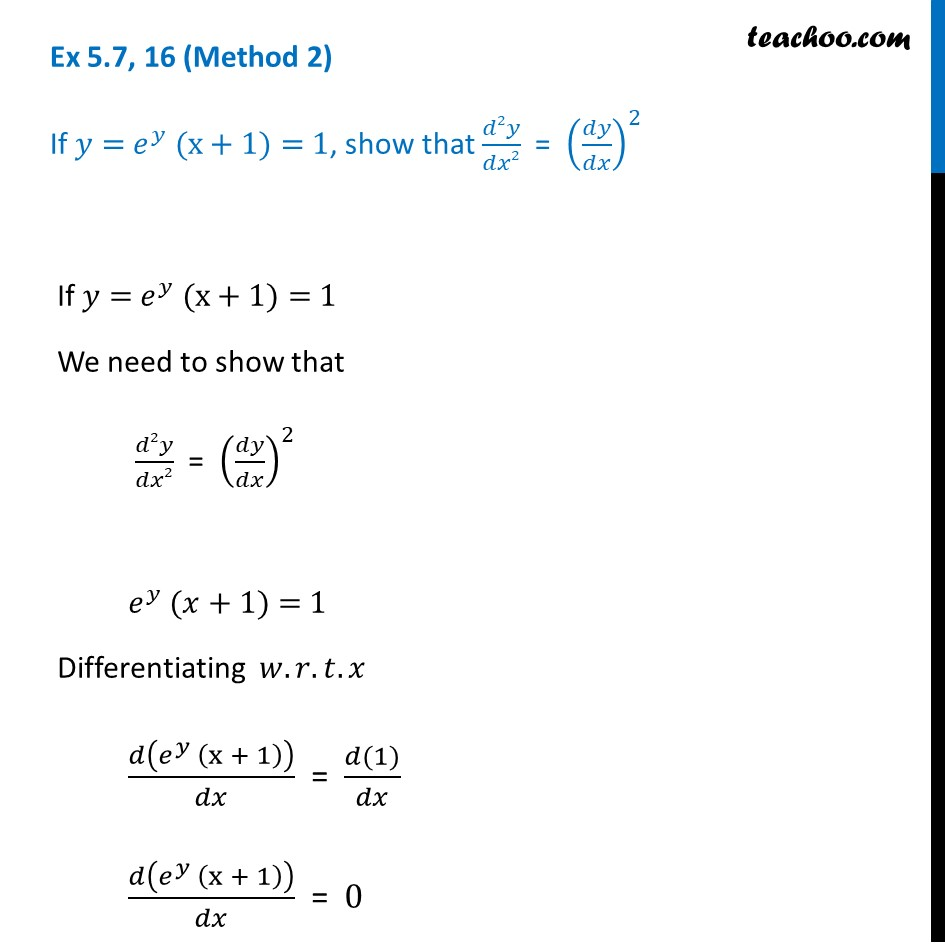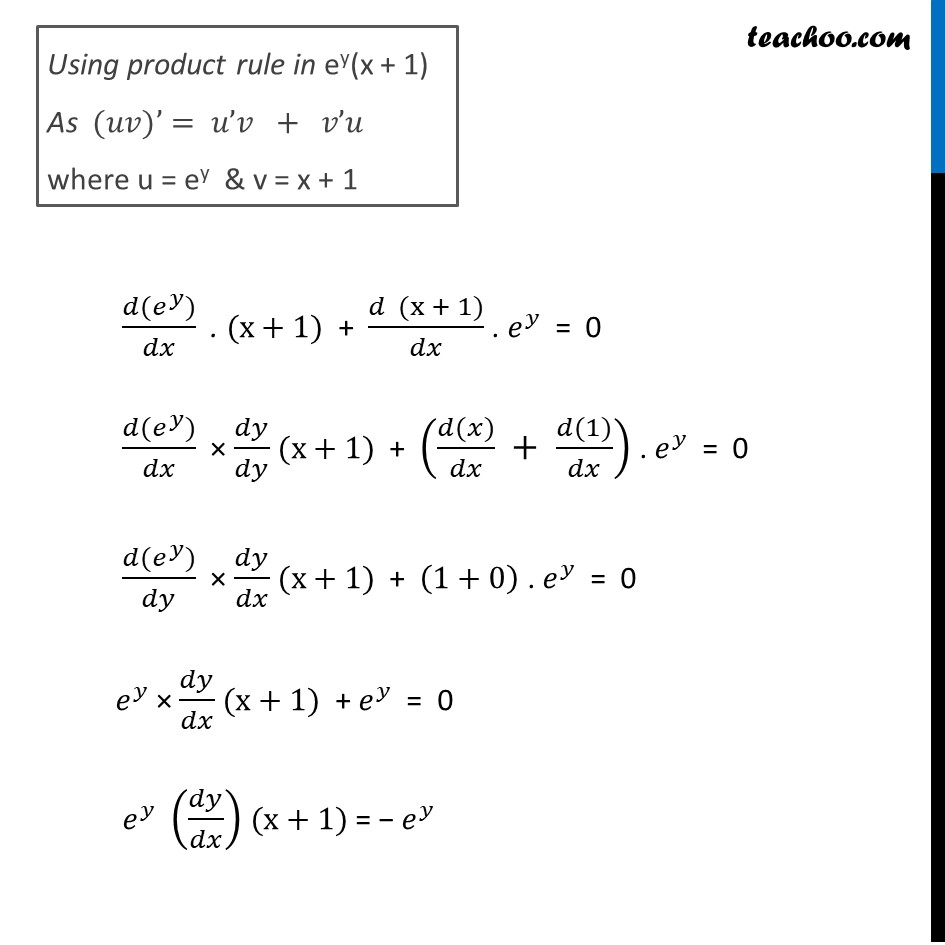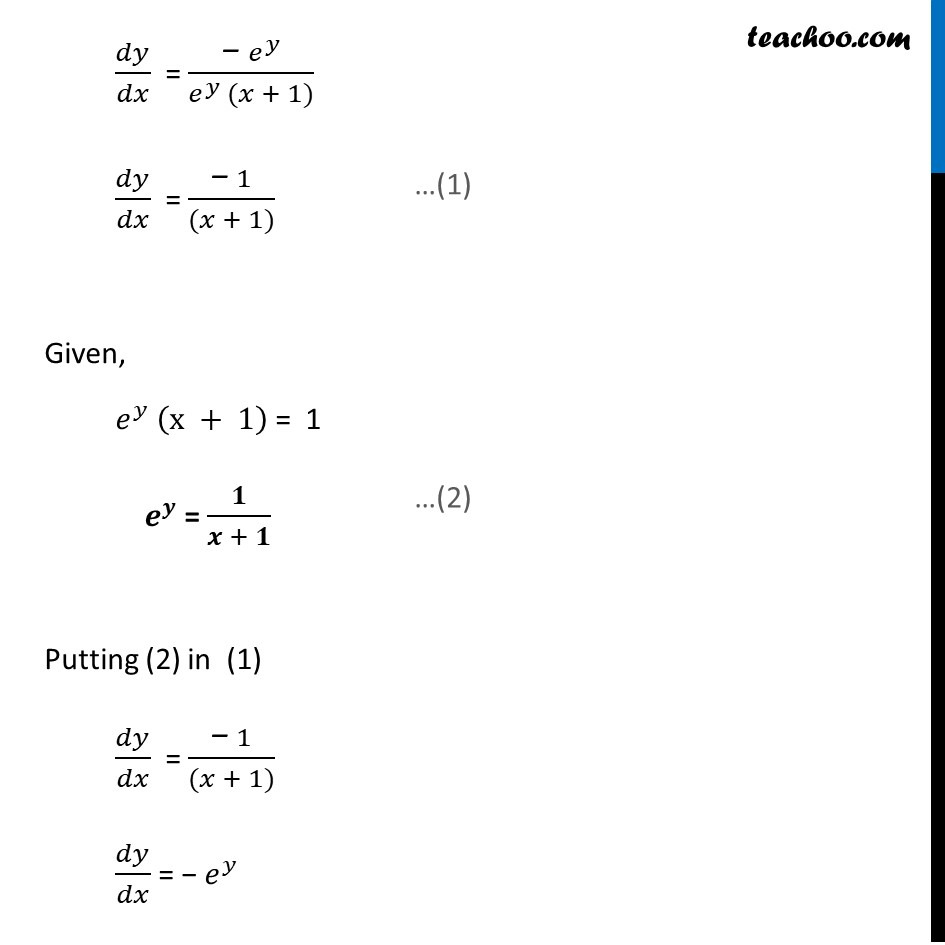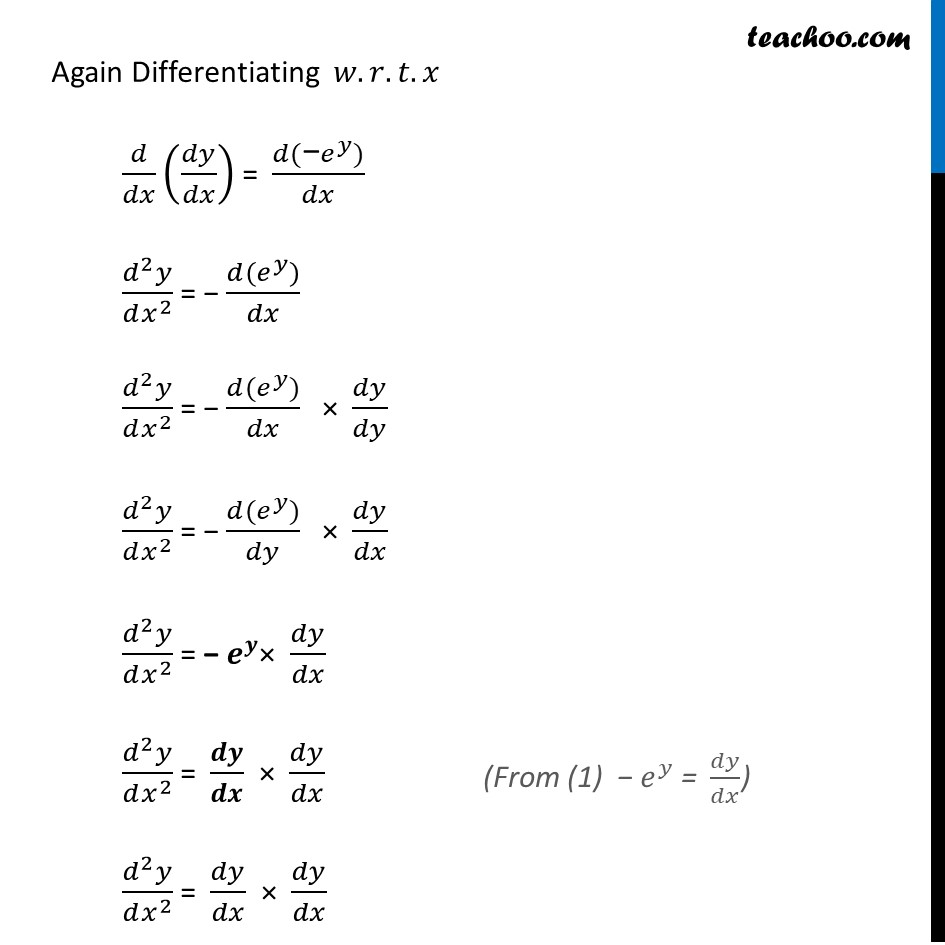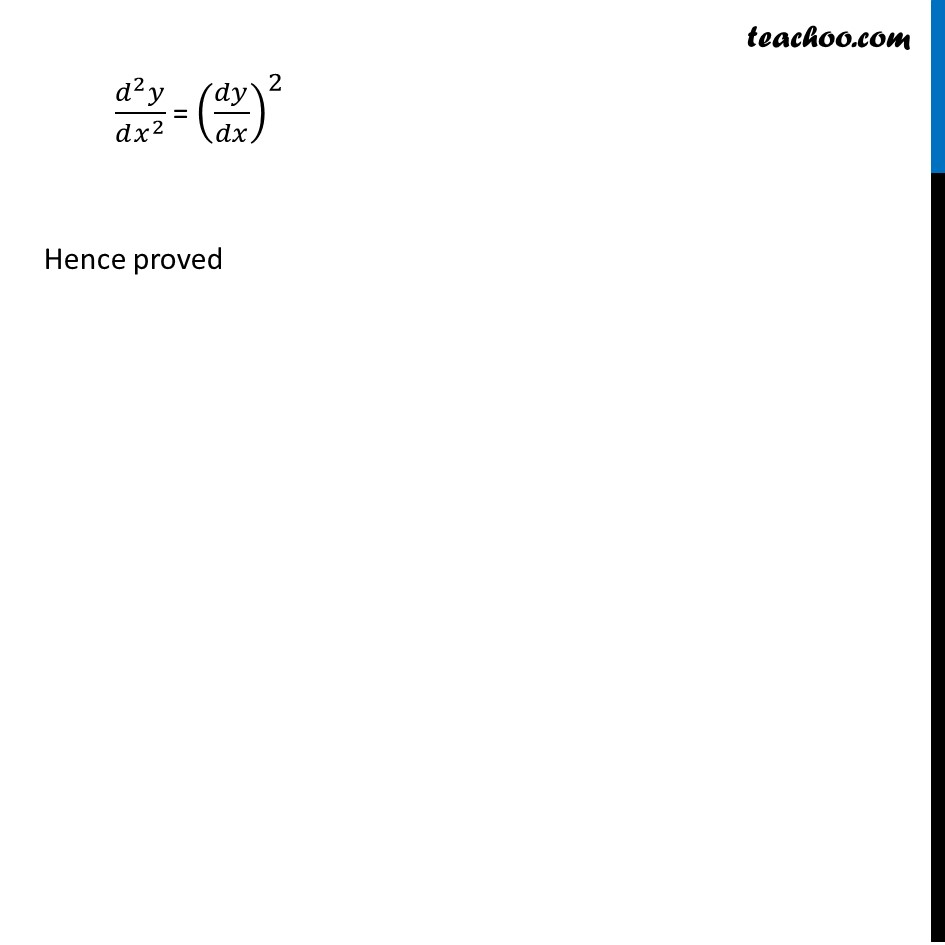Learn in your speed, with individual attention - Teachoo Maths 1-on-1 Class

### Transcript

Ex 5.7, 16 (Method 1) If 𝑒^𝑦 (x+1)= 1, show that 𝑑2𝑦/𝑑𝑥2 = (𝑑𝑦/𝑑𝑥)^2 We need to show that 𝑑2𝑦/𝑑𝑥2 = (𝑑𝑦/𝑑𝑥)^2 𝑒^𝑦 (x+1)= 1 Differentiating 𝑤.𝑟.𝑡.𝑥 𝑑(𝑒^𝑦 (x+1))/𝑑𝑥 = (𝑑(1))/𝑑𝑥 𝑑(𝑒^𝑦 (x + 1))/𝑑𝑥 = 0 Using product rule in ey(x + 1) As (𝑢𝑣)’= 𝑢’𝑣 + 𝑣’𝑢 where u = ey & v = x + 1 (𝑑(𝑒^𝑦))/𝑑𝑥 . (x+1) + (𝑑 (x + 1))/𝑑𝑥 . 𝑒^𝑦 = 0 (𝑑(𝑒^𝑦))/𝑑𝑥 × 𝑑𝑦/𝑑𝑦 (x+1) + ((𝑑(𝑥))/𝑑𝑥 + (𝑑(1))/𝑑𝑥) . 𝑒^𝑦 = 0 (𝑑(𝑒^𝑦))/𝑑𝑦 × 𝑑𝑦/𝑑𝑥 (x+1) + (1+0) . 𝑒^𝑦 = 0 𝑒^𝑦 × 𝑑𝑦/𝑑𝑥 (x+1) + 𝑒^𝑦 = 0 𝑒^𝑦 (𝑑𝑦/𝑑𝑥) (x+1) = − 𝑒^𝑦 𝑑𝑦/𝑑𝑥 = ("− " 𝑒^𝑦)/(𝑒^𝑦 (𝑥 + 1)) 𝑑𝑦/𝑑𝑥 = ("− " 1)/((𝑥 + 1)) Again Differentiating 𝑤.𝑟.𝑡.𝑥 𝑑/𝑑𝑥 (𝑑𝑦/𝑑𝑥) = 𝑑/𝑑𝑥 (("− " 1)/((𝑥+1) )) (𝑑^2 𝑦)/(𝑑𝑥^2 ) = −[((𝑑(1))/𝑑𝑥 . (𝑥 + 1) − 𝑑(𝑥 + 1)/𝑑𝑥 . 1)/〖(𝑥 + 1)〗^2 ] using Quotient Rule As, (𝑢/𝑣)^′= (𝑢’𝑣 − 𝑣’𝑢)/𝑣^2 where U = 1 & V = x + 1 = −[(0 . (𝑥+1) − 𝑑(𝑥+1)/𝑑𝑥 . 1)/〖(𝑥 + 1)〗^2 ] = −[(0 − (1 + 0) . 1)/〖(𝑥 + 1)〗^2 ] = −[(−1)/〖(𝑥 + 1)〗^2 ] = 1/〖(𝑥 + 1)〗^2 Hence (𝑑^2 𝑦)/(𝑑𝑥^2 ) = 1/〖(𝑥 + 1)〗^2 = ((−1)/( 𝑥 + 1))^2 = (𝑑𝑦/𝑑𝑥)^2 Hence proved Ex 5.7, 16 (Method 2) If 𝑦= 𝑒^𝑦 (x+1)= 1, show that 𝑑2𝑦/𝑑𝑥2 = (𝑑𝑦/𝑑𝑥)^2 If 𝑦= 𝑒^𝑦 (x+1)= 1 We need to show that 𝑑2𝑦/𝑑𝑥2 = (𝑑𝑦/𝑑𝑥)^2 𝑒^𝑦 (𝑥+1)= 1 Differentiating 𝑤.𝑟.𝑡.𝑥 𝑑(𝑒^𝑦 (x + 1))/𝑑𝑥 = (𝑑(1))/𝑑𝑥 𝑑(𝑒^𝑦 (x + 1))/𝑑𝑥 = 0 Using product rule in ey(x + 1) As (𝑢𝑣)’= 𝑢’𝑣 + 𝑣’𝑢 where u = ey & v = x + 1 (𝑑(𝑒^𝑦))/𝑑𝑥 . (x+1) + (𝑑 (x + 1))/𝑑𝑥 . 𝑒^𝑦 = 0 (𝑑(𝑒^𝑦))/𝑑𝑥 × 𝑑𝑦/𝑑𝑦 (x+1) + ((𝑑(𝑥))/𝑑𝑥 + (𝑑(1))/𝑑𝑥) . 𝑒^𝑦 = 0 (𝑑(𝑒^𝑦))/𝑑𝑦 × 𝑑𝑦/𝑑𝑥 (x+1) + (1+0) . 𝑒^𝑦 = 0 𝑒^𝑦 × 𝑑𝑦/𝑑𝑥 (x+1) + 𝑒^𝑦 = 0 𝑒^𝑦 (𝑑𝑦/𝑑𝑥) (x+1) = − 𝑒^𝑦 𝑑𝑦/𝑑𝑥 = ("− " 𝑒^𝑦)/(𝑒^𝑦 (𝑥 + 1)) 𝑑𝑦/𝑑𝑥 = ("− " 1)/((𝑥 + 1)) Given, 𝑒^𝑦 (x + 1) = 1 𝒆^𝒚 = 𝟏/(𝒙 + 𝟏) Putting (2) in (1) 𝑑𝑦/𝑑𝑥 = ("− " 1)/((𝑥 + 1)) 𝑑𝑦/𝑑𝑥 = − 𝑒^𝑦 …(1) …(2) Again Differentiating 𝑤.𝑟.𝑡.𝑥 𝑑/𝑑𝑥 (𝑑𝑦/𝑑𝑥) = (𝑑("−" 𝑒^𝑦))/𝑑𝑥 (𝑑^2 𝑦)/(𝑑𝑥^2 ) = − (𝑑(𝑒^𝑦))/𝑑𝑥 (𝑑^2 𝑦)/(𝑑𝑥^2 ) = − (𝑑(𝑒^𝑦))/𝑑𝑥 × 𝑑𝑦/𝑑𝑦 (𝑑^2 𝑦)/(𝑑𝑥^2 ) = − (𝑑(𝑒^𝑦))/𝑑𝑦 × 𝑑𝑦/𝑑𝑥 (𝑑^2 𝑦)/(𝑑𝑥^2 ) = "− " 𝒆^𝒚× 𝑑𝑦/𝑑𝑥 (𝑑^2 𝑦)/(𝑑𝑥^2 ) = 𝒅𝒚/𝒅𝒙 × 𝑑𝑦/𝑑𝑥 (𝑑^2 𝑦)/(𝑑𝑥^2 ) = 𝑑𝑦/𝑑𝑥 × 𝑑𝑦/𝑑𝑥 (From (1) "− " 𝑒^𝑦 " = " 𝑑𝑦/𝑑𝑥) (𝑑^2 𝑦)/(𝑑𝑥^2 ) = (𝑑𝑦/𝑑𝑥)^2 Hence proved